Home Practice
For learners and parents For teachers and schools
Textbooks
Full catalogue
Pricing SupportLog in

We think you are located in United States. Is this correct?

# Chapter 12: The particles that substances are made of

## 12.1 Atoms and compounds (ESADO)

### The atom as the building block of matter (ESADP)

We have seen that different materials have different properties. But what would we find if we were to break down a material into the parts that make it up (i.e. it's microscopic structure)? And how is it that this microscopic structure is able to give matter all its different properties?

The answer lies in the smallest building block of matter: the atom. It is the type of atoms, and the way in which they are arranged in a material, that affects the properties of that substance. This is similar to building materials. We can use bricks, steel, cement, wood, straw (thatch), mud and many other things to build structures from. The choice of atoms affects the properties of matter in the same way as the choice of building material affects the properties of the structure,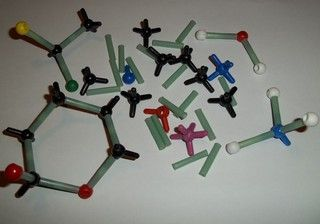It is not often that substances are found in atomic form (just as you seldom find a building or structure made from one building material). Normally, atoms are bonded (joined) to other atoms to form compounds or molecules. It is only in the noble gases (e.g. helium, neon and argon) that atoms are found individually and are not bonded to other atoms. We looked at some of the reasons for this in earlier chapters.

Compound

A compound is a group of two or more different atoms that are attracted to each other by relatively strong forces or bonds. The atoms are combined in definite proportions.

Compounds can be divided into molecular compounds (molecules), ionic compounds (salts) and metallic compounds (metals).

• Molecular compounds form as a result of covalent bonding where electrons are shared between non-metal atoms.

• Ionic compounds form as a result of ionic bonding where electrons are transferred from metals to non-metals.

• Metals are formed as a result of metallic bonding where metal atoms lose their outer electrons to form a lattice of regularly spaced positive ions and a “pool” of delocalised electrons that surround the positive ions.

The following diagram illustrates how compounds can be subdivided by the type of bonding and the structure:

#### Covalent molecular structures

Relatively small molecules are called covalent molecular structures. These exist and interact as separate molecules. Oxygen ($$\text{O}_{2}$$), water ($$\text{H}_{2}\text{O}$$), octane ($$\text{C}_{8}\text{H}_{18}$$), sulfur ($$\text{S}_{8}$$) and buckminsterfullerene ($$\text{C}_{60}$$, buckyballs) are all examples of covalent molecular structures.

#### Network structures

Compounds that exist as giant repeating lattice structures are called network structures. Examples include covalent molecules such as diamond, graphite and silica. Ionic substances are also network structures, for example a sodium chloride crystal is a huge lattice of repeating units made of sodium and chloride ions. All substances formed as a result of ionic bonding are network structures. Metals exist as large continuous lattice structures and are also classified as network structures. For example copper, zinc and iron can be seen as a giant crystals and are therefore considered to be network structures.

temp text

The structure of a molecule can be shown in many different ways. Sometimes it is easiest to show what a molecule looks like by using different types of diagrams, but at other times, we may decide to simply represent a molecule using its chemical formula or its written name.

#### Using formulae to show the structure of a molecule

A chemical formula is an abbreviated (shortened) way of describing a compound. In Chapter 2, we saw how chemical compounds can be represented using element symbols from the periodic table. A chemical formula can also tell us the number of atoms of each element that are in a compound and their ratio in that compound. If the compound is a covalent molecular compound then we can use the molecular formula.

Molecular formula

The molecular formula is a concise way of expressing information about the atoms that make up a particular covalent molecular compound. The molecular formula gives the exact number of each type of atom in the molecule.

For example in Figure 12.3 the molecular formula of 2-methyl propane is $$\text{C}_{4}\text{H}_{10}$$. This tells us that there are 4 carbon atoms and 10 hydrogen atoms in this molecule, i.e the ratio of carbon to hydrogen is $$4:10$$$$2:5$$empirical formula of the molecule.

The empirical and the molecular formulae can be the same. For example in carbon dioxide the molecular formula is $$\text{CO}_{2}$$. This is also the empirical formula since it is the simplest ratio.

Empirical formula

The empirical formula is a way of expressing the relative number of each type of atom in a chemical compound. The empirical formula does not show the exact number of atoms, but rather the simplest ratio of the atoms in the compound.

The empirical formula is useful when we want to write the formula for network structures. Since network structures may consist of millions of atoms, it is impossible to say exactly how many atoms are in each unit. It makes sense then to represent these units using their empirical formula. So, in the case of a metal such as copper, we would simply write $$\text{Cu}$$, or if we were to represent a unit of sodium chloride, we would simply write $$\text{NaCl}$$. Chemical formulae (i.e. the molecular or the empirical formula) therefore tell us something about the types of atoms that are in a compound and the ratio in which these atoms occur in the compound, but they don't give us any idea of what the compound actually looks like, in other words its shape. To show the shape of compounds we have to use diagrams. The simplest type of diagram that can be used to describe a compound is its structural formula. The structural formula for 2-methyl propane is shown in Figure 12.3.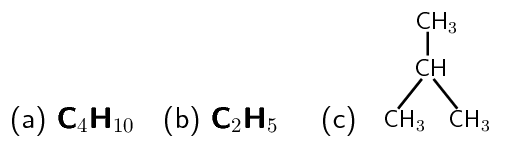Figure 12.3: Diagram showing (a) the chemical, (b) the empirical and (c) the structural formula of 2-methyl propane
temp text

#### Using diagrams to show the structure of a compound

Diagrams of compounds are very useful because they help us to picture how the atoms are arranged in the compound and they help us to see the shape of the compound. There are three types of diagrams that are commonly used:

• Wireframe or stick models

In this model, the bonds between atoms are shown as “sticks”. These “sticks” are coloured to show which atoms are bonding.

• Ball and stick models

This is a 3-dimensional molecular model that uses “balls” to represent atoms and “sticks” to represent the bonds between them. The centres of the atoms (the balls) are connected by straight lines which represent the bonds between them.

• Space-filling models

This is also a 3-dimensional molecular model. The atoms are represented by spheres.

Table 12.1 shows examples of the different types of models for all the types of compounds.

 Covalent molecular Covalent network Ionic network Metallic network Name of compound glucose graphite silver chloride zinc Formula $$\text{C}_{6}\text{H}_{12}\text{O}_{6}$$ or $$\text{CH}_{2}\text{O}$$ $$\text{C}$$ $$\text{AgCl}$$ $$\text{Zn}$$ Stick model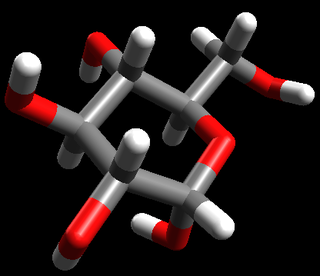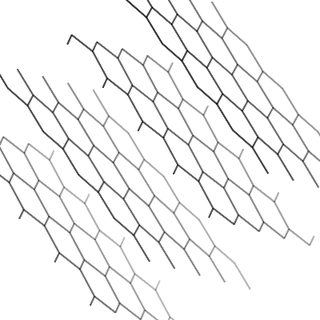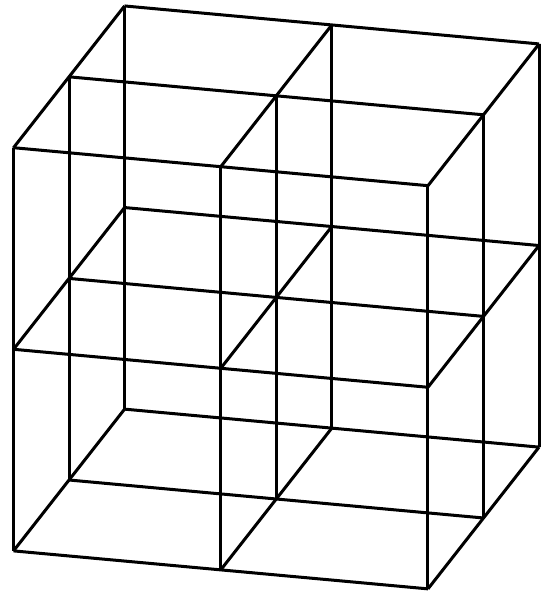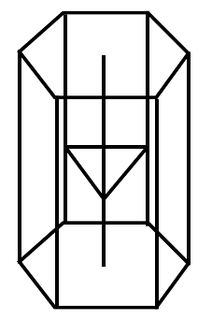Ball-and-stick model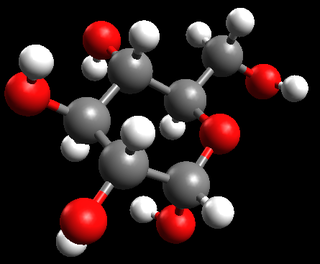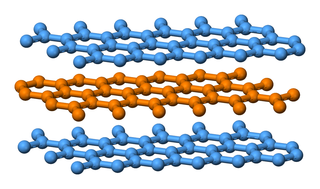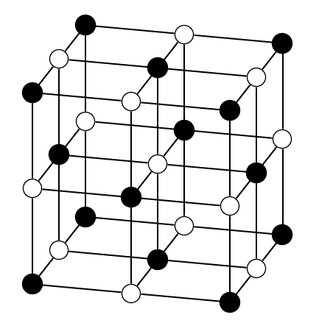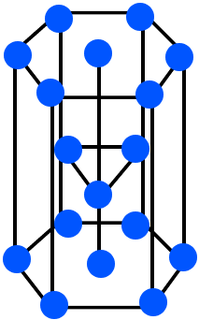Space-filling model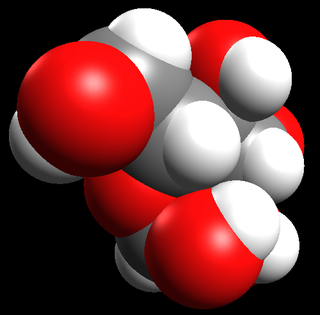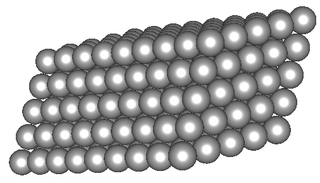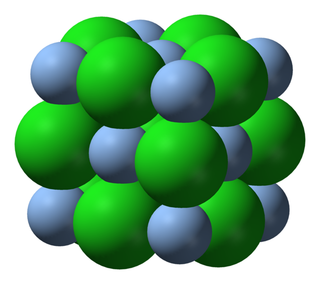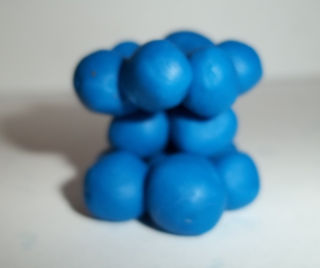Table 12.1: Different representations for compounds

## Representing compounds

A list of substances is given below. Make use of atomic model kits, play dough and toothpicks, or coloured polystyrene balls and skewer sticks to represent each of the substances in three dimensional structures.

• glucose ($$\text{C}_{6}\text{H}_{12}\text{O}_{6}$$)

• silica ($$\text{SiO}_{2}$$)

• sodium chloride ($$\text{NaCl}$$)

• sulfur ($$\text{S}_{8}$$)

• diamond ($$\text{C}$$)

• graphite ($$\text{C}$$)

• buckyballs($$\text{C}_{60}$$)

• sucrose ($$\text{C}_{12}\text{H}_{22}\text{O}_{11}$$)

• copper ($$\text{Cu}$$)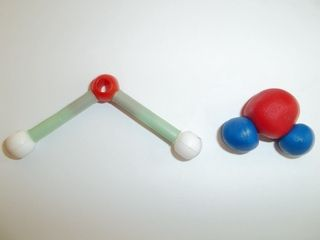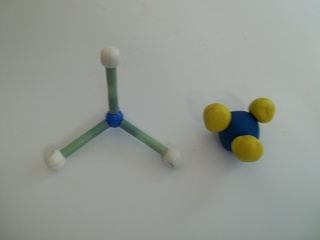temp text

CanvasMol (www.alteredqualia.com/canvasmol) is a website that allows you to view several compounds. You do not need to know these compounds, this is simply to allow you to see one way of representing compounds.

## Investigating elements and compounds

### Aim

To investigate three reactions to learn about elements and compounds.

### Apparatus

• Cal-C-Vita tablet

• test tubes

• Bunsen burner

• rubber stopper

• delivery tube

• lime water (a saturated solution of $$\text{Ca}(\text{OH})_{2}$$)

• candle

• matches

• copper sulfate ($$\text{CuSO}_{4}·5\text{H}_{2}\text{O}$$)

• zinc metal

• hydrochloric acid ($$\text{HCl}$$)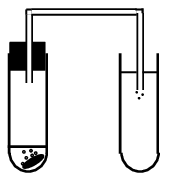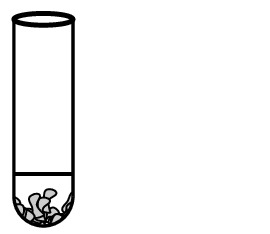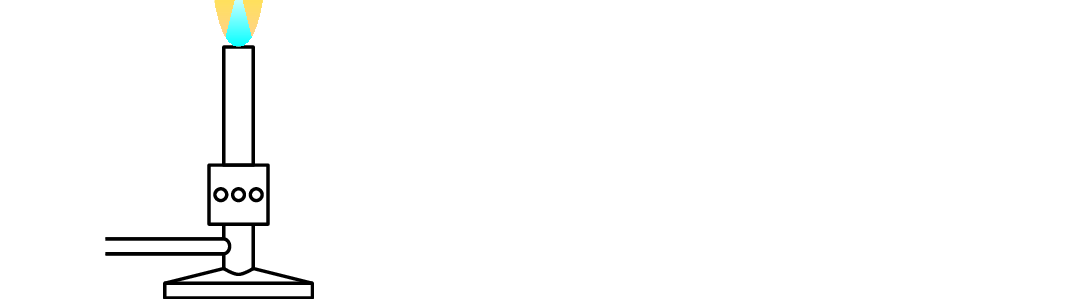### Method

Reaction 1

1. Pour clear lime water into a test tube.

2. Into a second test tube place a Cal-C-Vita tablet. Cover the tablet with water and immediately place a stopper and delivery tube into the test tube.

3. Place the other end of the delivery tube into the lime water in the first test tube. Allow it to bubble for 1-2 minutes.

4. Now remove the stopper from the second test tube and hold a lit candle at the mouth of the test tube.

Reaction 2

1. Place a few drops of zinc metal in a test tube and cover the pieces with dilute hydrochloric acid.

3. Now hold a burning match in the mouth of the test tube and observe what happens.

Reaction 3

1. Place a spatula full of copper sulfate crystals into a test tube and heat the tube over a Bunsen burner.

### Discussion and conclusion

In the first reaction the lime water goes milky due to the presence of carbon dioxide. (Lime water can be used to detect carbon dioxide gas.) The carbon dioxide gas comes from the sodium bicarbonate ($$\text{NaHCO}_{3}$$) in the tablet. When you hold the candle over the test tube, the carbon dioxide snuffs out the candle flame.

In the second reaction bubbles of hydrogen gas form. Zinc reacts with the hydrochloric acid to form zinc chloride and hydrogen gas.

In the third reaction the copper sulfate crystals go white and droplets of water form on the sides of the test tube. The copper sulfate crystals have lost their water of crystallisation.

## The electrolysis of water

### Aim

To investigate the elements that make up water.

### Apparatus

• water

• two pencils sharpened at both ends

• 9 volt battery

• connecting wire

• tape

• table salt or sodium sulfate

### Method

Set up the apparatus as shown above. Observe what happens.

### Results

You should observe bubbles forming at the tips of the pencils. Oxygen gas is formed at the positive side and hydrogen at the negative side.